# Class 12 Maths NCERT Solutions for Chapter 9 Differential Equations Exercise 9.5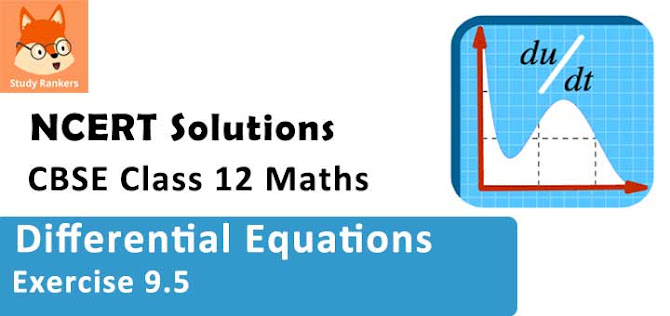### Differential Equations Exercise 9.5 Solutions

1. Show that the given differential equation is homogeneous and solve each of them
(x2 + xy) dy = (x2 + y2) dx

Solution

The given differential equation i.e. (x2 + xy) dy = (x2 + y2) dx can be written as :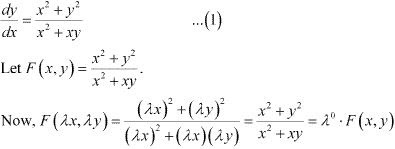This shows that equation (1) is a homogeneous equation.
To solve it, we make the substitution as :
y = vx
Differentiating both sides with respect to x, we get :
dy/dx = v + x(dv/dx)
Substituting the values of v and dy/dx in equation (1), we get :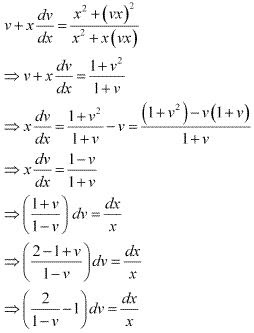Integrating both sides, we get :
-2 log (1 - v) - v = log x - log k
⇒ v = -2log (1 - v) - log x + log k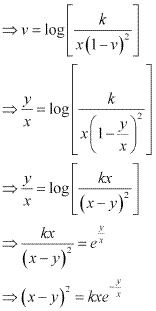This is the required solution of the given differential equation.

2. Show that the given differential equation is homogeneous and solve each of them
y' = (x + y)/x

Solution

The given differential equation is :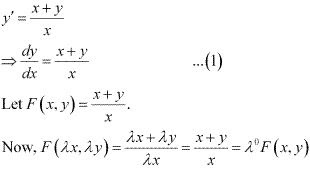Thus, the given equation is a homogeneous equation .
To solve it, we make the substitution as :
y = vx
Differentiating both sides with respect to x, we get :
dy/dx = V + x(dv/dx)
Substituting the values of y and dy/dx in equation (1), we  get :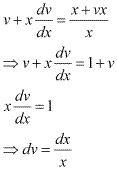Integrating both sides, we get :
v = log x + C
⇒ y/x = log x + C
⇒ y = x log x + Cx
This is the required solution of the given differential equation.

3. Show that the given differential equation is homogeneous and solve each of them
(x – y) dy – (x + y) dx = 0

Solution

The given differential equation is :
(x - y)dy - (x + y)dx = 0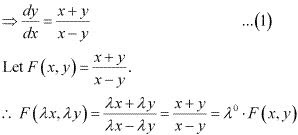Thus, the given differential equation is a homogeneous equation.
To solve it, we make the substitution as :
y = vx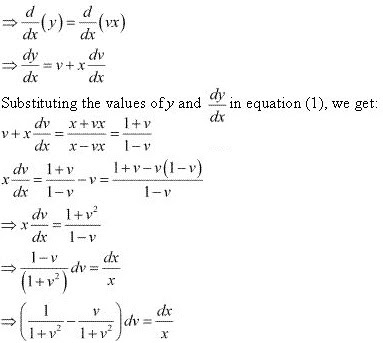Integrating both sides, we get :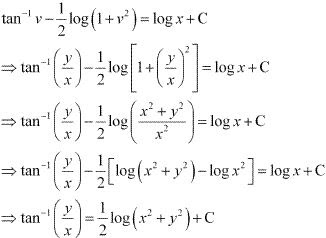This is the required solution of the given differential equation.

4. Show that the given differential equation is homogeneous and solve each of them
(x2 – y2) dx + 2xy dy = 0

Solution

The given differential equation is :
(x2 – y2) dx + 2xy dy = 0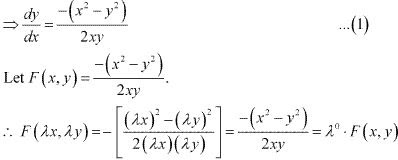Therefore, the given differential equation is a homogeneous equation.
To solve it, we make the substitution as :
y = vx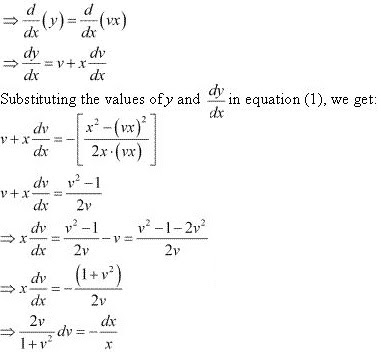Integrating both sides, we get :
log (1 + v)2 = -log x + log C = log (C/x)
⇒ 1 + v2 = C/x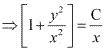⇒ x2 + y2 = Cx
This is the required solution of the given differential equation.

5. Show that the given differential equation is homogeneous and solve each of them
x2 (dy/dx) = x2 - 2y2 + xy

Solution

The given differential equation is :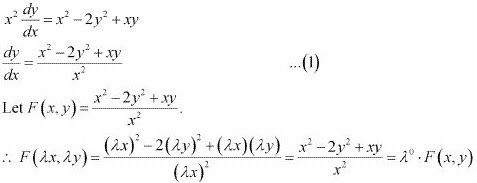Therefore, the given differential equation is a homogeneous equation.
To solve it, we make the substitution as :
y = vx
⇒ dy/dx = v + x(dv/dx)
Substituting the values of y and dy/dx in equation (1), we get :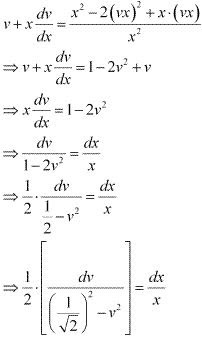Integrating both sides, we get: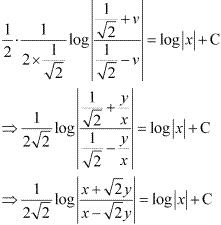This is the required solution for the given differential equation.

6. Show that the given differential equation is homogeneous and solve each of them
x dy -y dx = √(x2 + y2)dx

Solution

x dy -y dx =  √(x2 + y2 )dx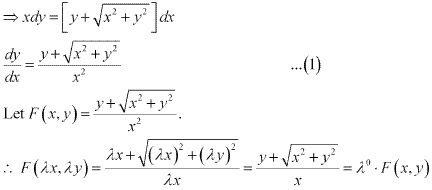Therefore, the given differential equation is a homogeneous equation.
To solve it, we make the substitution as :
y = vx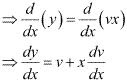Substituting the values of v and dy/dx in equation (1), we get :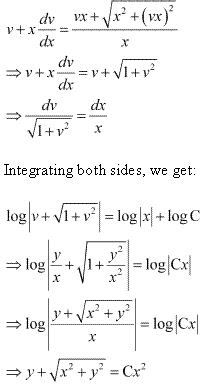This is the required solution of the given differential equation.

7. Show that the given differential equation is homogeneous and solve each of them
{x cos (y/x) + y sin (y/x)} ydx = {y sin (y/x) - x cos (y/x)} x dy

Solution

The given differential equation is  :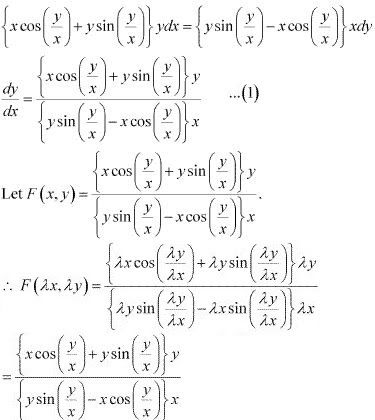= λ0. F(x, y)
Therefore, the given differential equation is a homogeneous equation.
To solve it, we make the substitution as :
y = vx
⇒ dy/dx = v + x = dv/dx
Substituting the values of y and dy/dx in equation (1), we get :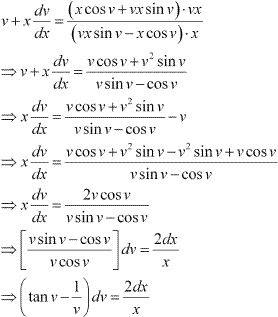Integrating both sides, we get :
log (sec v) - log v = 2 log x + log C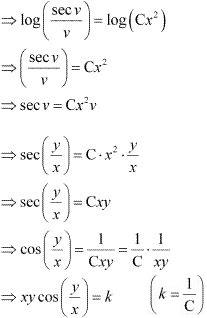This is the required solution of the given differential equation.

8. Show that the given differential equation is homogeneous and solve
x (dy/dx) - y + x sin (y/x) = 0

Solution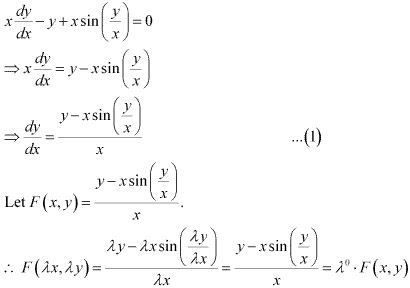Therefore, the given differential equation is a homogeneous equation.
To solve it, we make the substitution as :
y = vx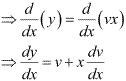Substituting the values of y and dy/dx in equation (1), we get :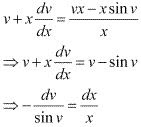⇒ cosec v dv = -dx/x
Integrating both sides, we get :
log|cosecv - cot v| = -log x + log C = log (C/x)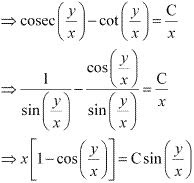This is the required solution of the given differential equation.

9. Show that the given differential equation is homogeneous and solve
y dx + x log (y/x)dy - 2xdy = 0
Solution
y dx + x log(y/x) dy - 2xdy = 0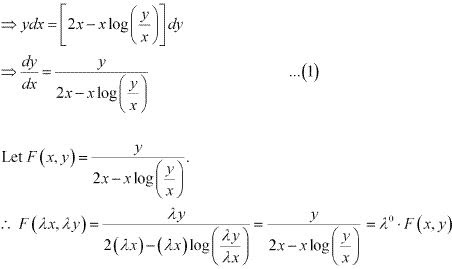Therefore, the given differential equation is a homogeneous equation.
To solve it, we make the substitution as :
y = vx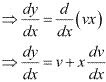Substituting the values of y and dy/dx in equation (1), we get: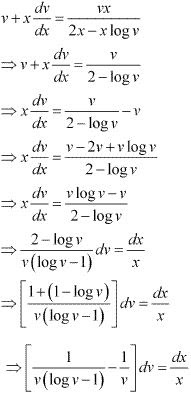Integrating both sides, we get :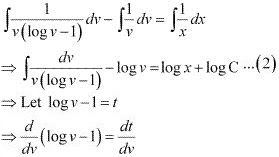⇒ 1/v = dt/dv
⇒ dv/v = dt
Therefore, equation (1) becomes :
⇒ ∫dt/t - log v = log x + log C
⇒ log t - log (y/x) = log (Cx)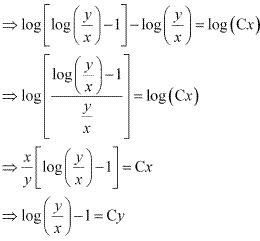This is the required solution of the given differential equation.

10. Show that the given differential equation is homogeneous and solve
(1 + ex/y ) dx + ex/y (1 - x/y)dy = 0
Solution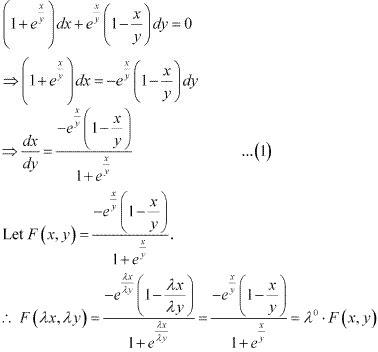Therefore, the given differential equation is a homogeneous equation.
To solve it, we make the substitution as :
x = vy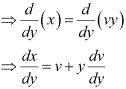Substituting the values of x and dx/dy in equation (1), we get :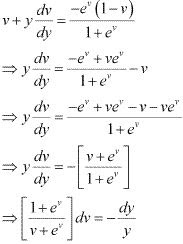Integrating both sides, we get :
⇒ log (v + ex ) = -log y + log C = log(C/y)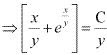⇒ x + yex/y = C
This is the required solution of the given differential equation.

11. For the differential equations find the particular solution satisfying the given condition:
(x + y) dy + (x – y) dx = 0; y = 1 when x = 1
Solution
(x + y)dy + (x - y)dx = 0
⇒ (x + y)dy = -(x - y) dx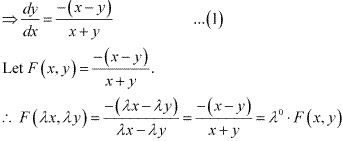Therefore, the given differential equation is a homogeneous equation.
To solve it, we make the substitution as :
y = vx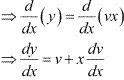Substituting the values of y and dy/dx in equation (1), we get :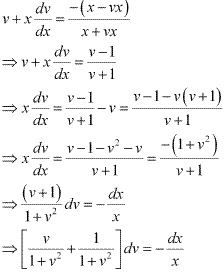Integrating both sides, we get :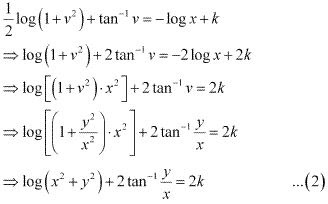Now, y = 1 at x = 1.
⇒ log 2 + 2 tan-11 = 2k
⇒ log 2 + 2 × π/4 = 2k
⇒ π/2 + log 2 = 2k
Substituting the value of 2k in equation (2), we get :
log(x2 + y2) + 2 tan-1(y/x) = π/2 + log 2
This is the required solution of the given differential equation.

12.For the differential equations find the particular solution satisfying the given condition:
x2 dy + (xy + y2) dx = 0; y = 1 when x = 1
Solution
x2 dy + (xy + y2 )dx = 0
⇒ x2 dy = -(xy + y2 )dx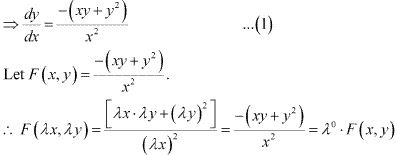Therefore, the given differential equation is a homogeneous equation.
To solve it, we make the substitution as :
y = vx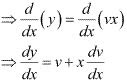Substituting the values of y and dy/dx in equation (1), we get: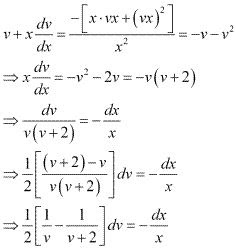Integrating both sides, we get :
(1/2) [log v - log(v + 2)] = -log x + log C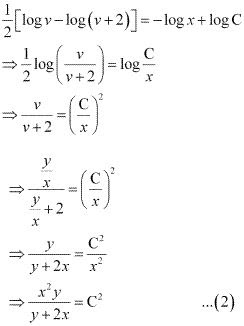Now, y =1 at x = 1.
⇒ 1/(1+2) = C2
⇒ C2 = 1/3
Substituting C2 = 1/3 in equation (2), we get:
x2 y/(y + 2x) = 1/3
⇒ y + 2x = 3x2 y
This is the required solution of the given differential equation.

13. For the differential equations find the particular solution satisfying the given condition:
[x sin2 (y/x - y)] dx + xdy = 0; y = π/4 when x = 1
Solution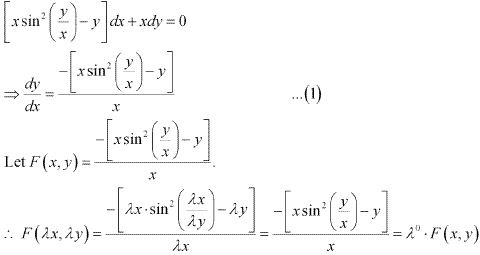Therefore, the given differential equation is a homogeneous equation.
To solve this differential equation, we make the substitution as :
y = vx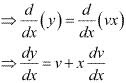Substituting the values of y and dy/dx in equation (1), we get :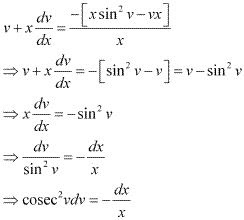Integrating both sides, we get :
- cot v = -log |x| - C
⇒ cot v = log |x| + C
⇒ cot(y/x) = log |x| + log C
⇒ cot (y/x) = log |Cx|  ...(2)
Now, y = π/4 at x = 1.
⇒ cot (π/4) = log |C|
⇒ 1 = log C
⇒ C = e1 = e
Substituting C = e in equation (2), we get :
cot (y/x) = log |ex|
This is the required solution of the given differential equation .

14. For the differential equations find the particular solution satisfying the given condition:
dy/dx - y/x + cosec(y/x) = 0; y = 0 when x = 1
Solution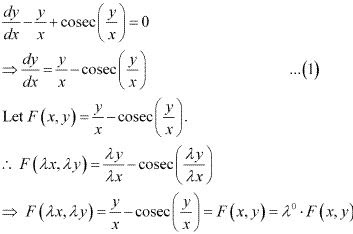Therefore, the given differential equation is a homogeneous equation.
To solve it, we make the substitution as :
y = vx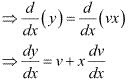Substituting the values of y and dy/dx in equation (1), we get :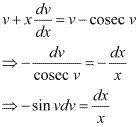Integrating both sides, we get :
cos v = log x + log C = log |Cx|
⇒ cos (y/x) = log |Cx| ...(2)
This is the required solution of the given differential equation.
Now, y = 0 at x = 1.
⇒ cos (0) = log C
⇒ 1 = log C
⇒ C = e1 = e
Substituting C = e in equation (2), we get :
cos(y/x) = log |(ex)|
This is the required solution of the given differential equation.

15. For the differential equations find the particular solution satisfying the given condition:
2xy + y2 - 2x2 (dy/dx) = 0; y = 2 when x = 1
Solution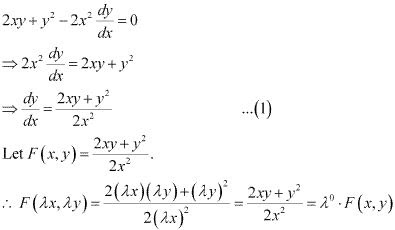Therefore, the given differential equation is a homogeneous equation.
To solve it, we make the substitution as :
y = vx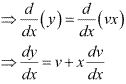Substituting the value of y and dy/dx in equation (1), we get :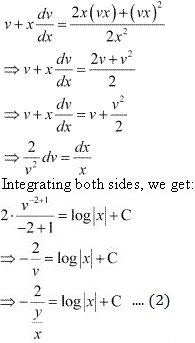Now, y = 2 at x = 1.
⇒ -1 = log (1) + C
⇒ C = -1
Substituting C = -1 in equation (2), we get :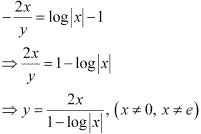This is the required solution of the given differential equation.

16. A homogeneous differential equation of the from dx/dt = h(x/y) can be solved by making the substitution
(A) y = vx
(B) v = yx
(C) x = vy
(D) x = v
Solution
For solving the homogeneous equation of the form dx/dy = h(x/y), we need to make the substitution as x = vy.
Hence, the correct answer is C.

17. Which of the following is a homogeneous differential equation?
(A) (4x + 6y + 5) dy – (3y + 2x + 4) dx = 0
(B) (xy) dx – (x3 + y3) dy = 0
(C) (x3 + 2y2) dx + 2xy dy = 0
(D) y2 dx + (x2 – xy – y2) dy = 0
Solution
Function F(x, y) is said to be the homogenous function of degree n, if
F(λx, λy) = λn F(x, y) for any non - zero constant (λ).
Consider the equation given in alternative D :
y2 dx + (x2 - xy - y2 )dy = 0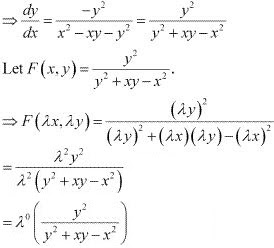Hence, the differential equation given in alternative D is a homogenous equation.